Test: Playing With Numbers

# Test: Playing With Numbers

Test Description

## 10 Questions MCQ Test NCERT Mathematics for CAT Preparation | Test: Playing With Numbers

Test: Playing With Numbers for Class 8 2022 is part of NCERT Mathematics for CAT Preparation preparation. The Test: Playing With Numbers questions and answers have been prepared according to the Class 8 exam syllabus.The Test: Playing With Numbers MCQs are made for Class 8 2022 Exam. Find important definitions, questions, notes, meanings, examples, exercises, MCQs and online tests for Test: Playing With Numbers below.
Solutions of Test: Playing With Numbers questions in English are available as part of our NCERT Mathematics for CAT Preparation for Class 8 & Test: Playing With Numbers solutions in Hindi for NCERT Mathematics for CAT Preparation course. Download more important topics, notes, lectures and mock test series for Class 8 Exam by signing up for free. Attempt Test: Playing With Numbers | 10 questions in 10 minutes | Mock test for Class 8 preparation | Free important questions MCQ to study NCERT Mathematics for CAT Preparation for Class 8 Exam | Download free PDF with solutions
 1 Crore+ students have signed up on EduRev. Have you?
Test: Playing With Numbers - Question 1

### Substitute digits for the letter A to make the following Addition problem true.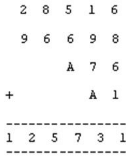Detailed Solution for Test: Playing With Numbers - Question 1

Explanation : 1+9+7 +4 so the answer will 23 and if we add 5+6+4 so the answer will 17.

Test: Playing With Numbers - Question 2

### Substitute digits for the letter N to make the following Addition problem true.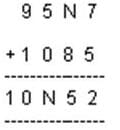Detailed Solution for Test: Playing With Numbers - Question 2

We have 7+5=12, 1 is carried forward, so 1+N+8=_5, which gives N=6

Test: Playing With Numbers - Question 3

### Substitute digits for the letter A to make the following Addition problem true.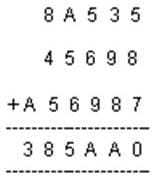Detailed Solution for Test: Playing With Numbers - Question 3

Adding one’s place 5+7+8=20, 2 is carried forward.adding the ten’s place 2+3+9+8=22, this means A=2

Test: Playing With Numbers - Question 4

Substitute digits for the letter A to make the following Multiplication problem true.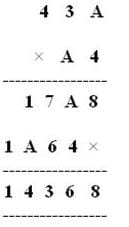Detailed Solution for Test: Playing With Numbers - Question 4

We have A*4=_8 so A can be 2 or 7.now if A is 7,then we have 2 as carried forward so 4*3+2=14_7A,when A=2, we have no carried forward so4*3=12=_2=A. Hence A=2

Test: Playing With Numbers - Question 5

Substitute digits for the letters P and Q to make the following Multiplication problem true.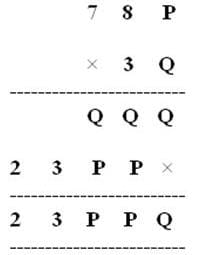Detailed Solution for Test: Playing With Numbers - Question 5

As we see the addition part,we have 23PPQ, this means that we don’t have any carried forward for the thousand’s place this means we have only single digit by adding Q and P, so Q+P =P only when Q=0,and now seeing the multiplication we have 3*P=_P which is only possible when P=5.

Test: Playing With Numbers - Question 6

By which of the following number 168 is divisible?

Detailed Solution for Test: Playing With Numbers - Question 6

The numbers ending with 2,4,6,8 ,0 are divisible by 2.

Test: Playing With Numbers - Question 7

Substitute digits for the letters A and B to make the following Multiplication problem true.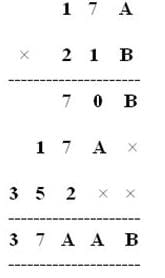Detailed Solution for Test: Playing With Numbers - Question 7

Looking at the addition of the hundred’s place we have 7+7+2=_A which gives A=6. Coming back to the multiplication which is A*B=B where A=6 ,so B can be 4 or 6, but when you take B=6, we have 3 as carried forward and 7*6+3 is not equal to 0. Hence B=4 and A=6.

Test: Playing With Numbers - Question 8

By which of the following number 225 is divisible? 2, 3, 4, and 6

Test: Playing With Numbers - Question 9

Suppose that the division N ÷ 5 leaves a remainder of 4, and the division N ÷ 2 leaves a remainder of 1. What must be the one’s digit of N?

Detailed Solution for Test: Playing With Numbers - Question 9

Since N leaves the remainder of 4 when divided by 5. the possible values in ones place of number N are 4 or 9.

now, since it leaves a remainder of 1 when divided by 2, the N would be an odd number. hence ones digit of N is also an odd number. which means ones digit of our number N is 9.

Test: Playing With Numbers - Question 10

If a number is divisible by 9, then it is also divisible by which number?

Detailed Solution for Test: Playing With Numbers - Question 10 Yes correct answer is 3 because 9 is divisible by 3 ... 3×3=9..

## NCERT Mathematics for CAT Preparation

276 docs|149 tests
 Use Code STAYHOME200 and get INR 200 additional OFF Use Coupon Code
Information about Test: Playing With Numbers Page
In this test you can find the Exam questions for Test: Playing With Numbers solved & explained in the simplest way possible. Besides giving Questions and answers for Test: Playing With Numbers, EduRev gives you an ample number of Online tests for practice

## NCERT Mathematics for CAT Preparation

276 docs|149 tests